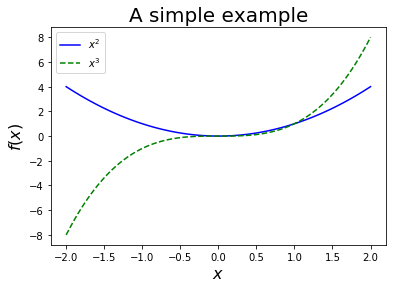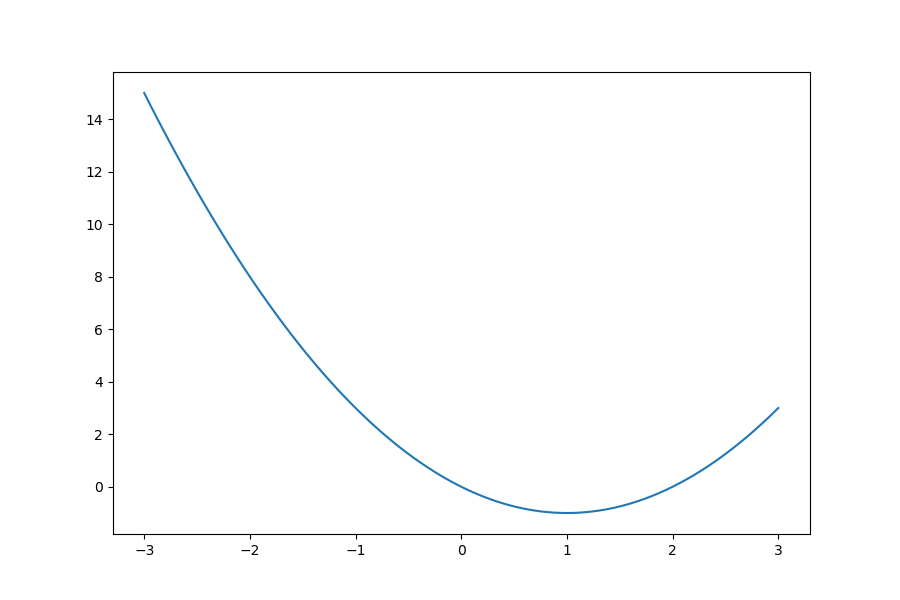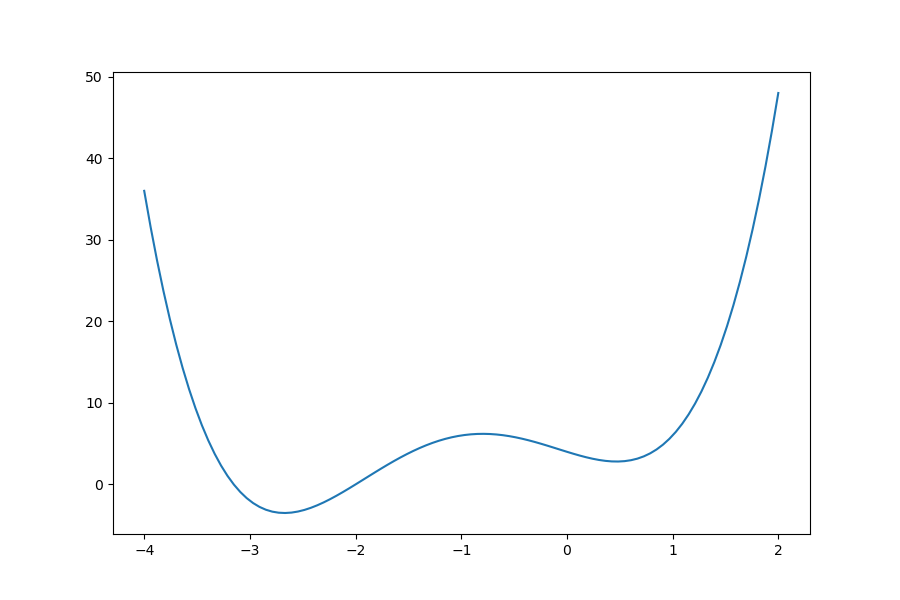# Introduction to Python for Scientific Computing - Tutorial n°3¶

Mohammed Ait Lahcen, Economic Theory Group, Faculty of Business and Economics, University of Basel

In this tutorial we take a look at three of the most important scientific packages in Python: NumPy, Matplotlib and Scipy.

For a more detailed introduction to Python for scientific computing you can refer to the lectures available at QuantEcon.org.

## Import statements and packages¶

Since Python is a general purpose language, the core language is quite small for practical reasons (easier maintainance and learning). Most of the "advanced" functionalities are created by developers as separate packages (also called modules or libraries) that must be installed and imported in order to be used. This is in particular the case for scientific computing in Python.

For example, you can import the Numpy package by writing:

In :
import numpy

numpy.sqrt(16)

Out:
4.0

The next way of doing is the "standard" way:

In :
import numpy as np

np.sqrt(16)

Out:
4.0

Another way of importing the sqrt function could be:

In :
from numpy import sqrt

sqrt(16)

Out:
4.0

The advantage of the third method is less typing if sqrt is used often in the code. However, in a long program, it’s hard for the reader to know which package the function sqrt has been imported from. This can be important if similarly named functions are available from several packages.

Using * instead of sqrt will import all of the content of Numpy. It is highly recommended to avoid doing this because it imports lots of variable names which can be a potential source of conflict.

## Numpy¶

Numpy is the fundamental package for scientific computing with Python. It offers a powerful N-dimensional array object, sophisticated array operations and functions, tools for integrating C/C++ and Fortran code, useful linear algebra, Fourier transform, and random number capabilities.

We start by importing Numpy into the global namespace (if it's not aleady there):

In :
import numpy as np


### Math with Numpy¶

Numpy offers a set of highly optimized standard math functions:

In :
x = 5

np.exp(x)

Out:
148.4131591025766
In :
y = -3

np.abs(y)

Out:
3
In :
np.pi

Out:
3.141592653589793
In :
np.e

Out:
2.718281828459045

### Arrays¶

Numpy’s main object is the homogeneous multidimensional array numpy.ndarray. An array is a table of elements (usually numbers), all of the same type, indexed by a tuple of positive integers. In Numpy dimensions are called axes. The number of axes is called rank.

Numpy arrays are less flexible but much more memory efficient and faster to access compared to Python lists.

In :
a = np.zeros(3)
a

Out:
array([0., 0., 0.])
In :
type(a)

Out:
numpy.ndarray

The most important attributes of an ndarray object are:

• ndarray.dtype : data type of the elements in the array.
• ndarray.ndim : the number of axes (dimensions) of the array.
• ndarray.shape : the dimensions or rank of the array (size of the array in each dimension).
• ndarray.size : the total number of elements of the array.

One-dimensional arrays are printed as rows, bidimensionals as matrices and tridimensionals as lists of matrices...

In :
a = np.arange(15)
a

Out:
array([ 0,  1,  2,  3,  4,  5,  6,  7,  8,  9, 10, 11, 12, 13, 14])
In :
a.dtype

Out:
dtype('int32')
In :
a = a.reshape(3, 5)
a

Out:
array([[ 0,  1,  2,  3,  4],
[ 5,  6,  7,  8,  9],
[10, 11, 12, 13, 14]])
In :
a.shape

Out:
(3, 5)
In :
a.ndim

Out:
2
In :
a.size

Out:
15
In :
len(a)

Out:
3

### Array creation¶

There are several ways to create arrays. You can create an empty array:

In :
a = np.empty(5)
a

Out:
array([0.00000000e+000, 6.95311186e-310, 1.48219694e-323,             nan,
5.34041153e-307])

You can also create a regular grid:

In :
a = np.linspace(-1,1,11)  # Specifies the grid size
a

Out:
array([-1. , -0.8, -0.6, -0.4, -0.2,  0. ,  0.2,  0.4,  0.6,  0.8,  1. ])
In :
a = np.arange(-1,1,0.2)  # Specifies the grid step, excludes the upper bound
a

Out:
array([-1.00000000e+00, -8.00000000e-01, -6.00000000e-01, -4.00000000e-01,
-2.00000000e-01, -2.22044605e-16,  2.00000000e-01,  4.00000000e-01,
6.00000000e-01,  8.00000000e-01])

You can also create arrays of zeros or ones:

In :
a = np.ones(3)
a

Out:
array([1., 1., 1.])
In :
a = np.identity(3)
a

Out:
array([[1., 0., 0.],
[0., 1., 0.],
[0., 0., 1.]])

and arrays of random numbers:

In :
a = np.random.rand(3,2)
a

Out:
array([[0.79961152, 0.45606796],
[0.41030638, 0.16802722],
[0.65638152, 0.93569703]])

NumPy arrays can be made from regular Python sequences (lists or tuples). np.array transforms sequences of sequences into two-dimensional arrays, sequences of sequences of sequences into three-dimensional arrays, and so on.

In :
b = np.array([[1,2,3], [4,5,6]])
b

Out:
array([[1, 2, 3],
[4, 5, 6]])

np.array accepts a single list (of lists) of numbers as an argument and not multiple numeric arguments:

In :
a = np.array(1,2,3,4)    # WRONG
a

---------------------------------------------------------------------------
ValueError                                Traceback (most recent call last)
----> 1 a = np.array(1,2,3,4)    # WRONG
2 a

ValueError: only 2 non-keyword arguments accepted
In :
a = np.array([1,2,3,4])  # RIGHT
a

Out:
array([1, 2, 3, 4])

### Array indexing¶

In :
a = np.linspace(1,5,5)
a

Out:
array([1., 2., 3., 4., 5.])

One-dimensional array indexing is similar to list indexing:

In :
a

Out:
1.0

To access the last element use

In :
a[-1]

Out:
5.0

a[:] : returns a copy of the whole array

In :
a[:]

Out:
array([1., 2., 3., 4., 5.])

a[start:] : returns elements from start to the rest of the array

In :
a[2:]

Out:
array([3., 4., 5.])

a[:end] : returns elements from the beginning through end-1

In :
a[:5]

Out:
array([1., 2., 3., 4., 5.])

a[start:end] : returns elements from start through end-1

In :
a[2:4]

Out:
array([3., 4.])

Indexing multi-dimensional arrays is different from list indexing:

In :
b = np.array([[1, 2], [3, 4]])
b

Out:
array([[1, 2],
[3, 4]])
In :
b

Out:
array([1, 2])

or

In :
b[0,:]

Out:
array([1, 2])

Slicing along the second dimension:

In :
b[:,1]

Out:
array([2, 4])

To index an individual element, we have to provide the coordinates:

In :
b[1,0]

Out:
3

### Array methods¶

The ndarray data type offers several methods:

In :
a = np.array([3, 0, 15, 5])
a

Out:
array([ 3,  0, 15,  5])
In :
a.sort()
a

Out:
array([ 0,  3,  5, 15])
In :
a.sum()

Out:
23
In :
a.min()

Out:
0
In :
a.mean()

Out:
5.75
In :
a.var()

Out:
31.6875
In :
a.shape = (2,2)
a

Out:
array([[ 0,  3],
[ 5, 15]])
In :
a.T

Out:
array([[ 0,  5],
[ 3, 15]])

### Array operations¶

Arithmetic operations apply element-wise:

In :
a = np.array([0,1,2,3])
b = np.array([4,5,6,7])

In :
a + b

Out:
array([ 4,  6,  8, 10])
In :
a * b

Out:
array([ 0,  5, 12, 21])
In :
a - 4

Out:
array([-4, -3, -2, -1])
In :
a.shape = (2,2)
b.shape = (2,2)

In :
a

Out:
array([[0, 1],
[2, 3]])
In :
b

Out:
array([[4, 5],
[6, 7]])

Element-wise multiplication:

In :
a * b

Out:
array([[ 0,  5],
[12, 21]])

For matrix multiplication, you can either use the @ operator:

In :
a @ b

Out:
array([[ 6,  7],
[26, 31]])

or the array dot method

In :
a.dot(b)

Out:
array([[ 6,  7],
[26, 31]])

or the Numpy dot product function:

In :
np.dot(a,b)

Out:
array([[ 6,  7],
[26, 31]])

Numpy math functions are optimized to work directly on arrays:

In :
np.exp(a)

Out:
array([[ 1.        ,  2.71828183],
[ 7.3890561 , 20.08553692]])
In :
np.sin(a)

Out:
array([[0.        , 0.84147098],
[0.90929743, 0.14112001]])

## Matplotlib¶

There are many powerful possibilities when it comes to data vizualisation in Python (Matplotlib, Seaborn, Bokeh, ggplot, etc). The one mostly used for non-interactive 2D and 3D plots is Matplotlib. The Matplotlib library is similar to Matlab's plotting interface.

In :
import matplotlib.pyplot as plt


We first generate two arrays to plot:

In :
x = np.linspace(-2, 2, 100)
y = x ** 2
z = x ** 3

In :
plt.figure()
plt.plot(x,y,'-b',label = r'$x^2$')
plt.plot(x,z,'--g',label = r'$x^3$')
plt.legend(loc='best')
plt.title('A simple example',fontsize='20')
plt.ylabel(r'$f(x)$',fontsize='16')
plt.xlabel(r'$x$',fontsize='16')
plt.show()
#fig.savefig('foo.pdf')The Jupyter magic command %matplotlib enables the choice of the plotting backend. To list all available backends type:

In :
%matplotlib --list

Available matplotlib backends: ['tk', 'gtk', 'gtk3', 'wx', 'qt4', 'qt5', 'qt', 'osx', 'nbagg', 'notebook', 'agg', 'svg', 'pdf', 'ps', 'inline', 'ipympl', 'widget']


The backend notebook allows for interactive figures inside the notebook:

In :
%matplotlib notebook


## SciPy¶

SciPy is the main stack of scientific libraries for Python. It is built on top of Numpy and includes routines for almost everything you might need in your computational economics and finance work:

• Optimization and root finding
• Probability distributions
• Numerical integration and differentiation
• Function interpolation
• Linear algebra
• ...

You can find a list of all SciPy subpackages in this online tutorial.

In what follows, we will limit ourselves to exploring the use of the numerical optimization subpackage scipy.optimize which provides several commonly used root-finding and optimization algorithms. A detailed list is available here.

Note that the common practice when using Scipy is to import the required subpackages indiviually instead of importing all of Scipy.

### Root finding¶

The function root offers a simple interface to several root finding methods. In order to use it, we first define the objective function, then pass it to root along with an initial guess of the roots.

Let's give it a try with the following simple quadratic equation: $$x^2 - 2 x = 0$$

In :
def f(x):
"""
The objective function

"""

return x ** 2 - 2 * x


Let's first plot the function to get a better understanding of its behavior:

In :
x = np.linspace(-3,3,100)

plt.figure(figsize=(9,6))
plt.plot(x,f(x))
plt.show()From the figure above we can see that the equation has two roots $0$ and $2$. Now let's solve numerically for these roots:

In :
from scipy.optimize import root

root(f,[-3,4],method='hybr')

Out:
    fjac: array([[ 0.99998618,  0.00525799],
[-0.00525799,  0.99998618]])
fun: array([ 1.35315498e-13, -2.66453526e-13])
message: 'The solution converged.'
nfev: 15
qtf: array([ 1.92194663e-10, -4.85040307e-10])
r: array([-1.99471838,  0.00784877,  2.00530717])
status: 1
success: True
x: array([-6.76577491e-14,  2.00000000e+00])

As expected, the solver returns 0 and 2 as the roots of the objective function.

Notice that we had to provide two initial guesses, that is one for each root. Had we provided one initial guess the solver would have returned only one root.

In order to access the results provided by the solver we can just store them in a variable and then call the appropriate field. For example:

In :
sol = root(f,[-3,4],method='hybr')

sol.x

Out:
array([-6.76577491e-14,  2.00000000e+00])
In :
sol.success

Out:
True

Choosing the right root finding method will depend on the nature of the problem at hand. The list of all the methods accessible through root can be found here.

The function root can also be used to solve systems of linear and non-linear equations: \begin{align} x^2 + y^2 &= 3 \\ x - 3\log{y} &= 1 \end{align}

For that, we have to write the equations passed to the root-finding solver in the form $f(x,y) = 0$:

In :
def g(x):
return [x**2 + x**2 - 3, x - 3*np.log(x) -1]

In :
sol = root(g,[1,1],method='lm')

sol.x

Out:
array([1.3257028 , 1.11468026])

### Optimization¶

scipy.optimize includes a number of the most common optimization algorithms (also called solvers or routines) used to find the extrema of a user-defined objective function. Fortunately, Scipy offers a standardized interface or "wrapper" to use those solvers called minimize. The list of algorithms available through minimize can be found here.

Notice that most optimization solvers, including the ones in Scipy, allow to only minimize the objective function. However, this is not an issue since maximizing $f$ is equivalent to minimizing $-f$.

#### Unconstrained Optimization¶

We want to minimize the following function: $$f(x) = 4x^3 + (x -2)^2 + x^4$$

In :
def f(x):

return 4 * x ** 3 + (x-2) ** 2 + x ** 4


Again, we plot the function and see how it behaves:

In :
x = np.linspace(-4, 2, 100)

plt.figure(figsize=(9,6))
plt.plot(x, f(x))
plt.show()As you can see, the function has two local minima of which one is global. Depending on the solver we choose and the initial guess we provide, the solver may or may not get "stuck" at the local minimum:

In :
from scipy.optimize import minimize

sol.x

Out:
array([0.46962891])
In :
sol = minimize(f, x0=2, method='CG')

sol.x

Out:
array([-2.67298166])

If we don’t know the neighborhood of the global minimum to choose the initial point, we need to resort to costlier global optimization methods. To find the global minimum, the simplest algorithm is the brute force algorithm brute, in which the function is evaluated on each point of a given grid. We can also provide a finish optimizer which will take the solution of brute as an initial guess and try to “polish” it, i.e. seek a more precise (local) minimum near the gridpoint returned by brute:

In :
from scipy.optimize import brute

r = (-4,2)

sol_global = brute(f,ranges=(r,),finish=None)

sol_global

Out:
-2.736842105263158
In :
sol_global = brute(f,ranges=(r,),finish=minimize)

sol_global

Out:
array([-2.67298164])

#### Constrained Optimization¶

We consider the following maximization problem: $$\max_{x,y} \, \, 3xy - x^3$$ subject to the following constraints: \begin{align} 2x - y &= -5 \\ 5x + 2y &\geq 37 \\ x &\geq 0 \\ y &\geq 0 \end{align}

To solve this problem we use minimize with the method SLSQP which accepts both equality and inequality constraints as well as interval bounds on the choice variables:

In :
def g(x):

return - (3 * x * x - x ** 3)


The constraints are defined as a sequence of dictionaries, with keys type, fun and jac. The constraints can be passed as normal or lambda functions.

Bounds for the choice variables $x$ (only for L-BFGS-B, TNC and SLSQP) have to be defined as (min, max) pairs for each element in $x$. You can use None or np.inf for one of min or max when there is no bound in that direction.

Method-specific options such as the maximum number of iterations or convergence criteria can be supplied through the options dict.

In :
from scipy.optimize import minimize

cons = ({'type': 'eq', 'fun': lambda x: 2*x - x + 5},
{'type': 'ineq', 'fun': lambda x: 5*x + 2*x -37})

bnd = [(0, np.inf),(0,np.inf)]

sol = minimize(g, x0=[0, 0], method='SLSQP', constraints=cons,  bounds=bnd, options={'disp': True,'ftol': 1e-10})

sol.x

Optimization terminated successfully.    (Exit mode 0)
Current function value: -100.0
Iterations: 8
Function evaluations: 33

array([ 5.00000001, 15.00000003])